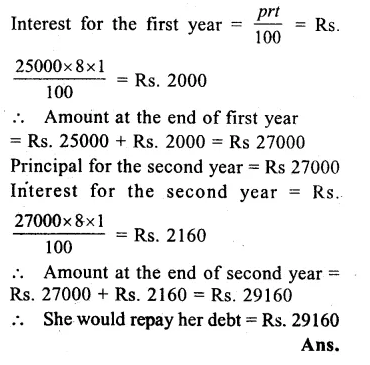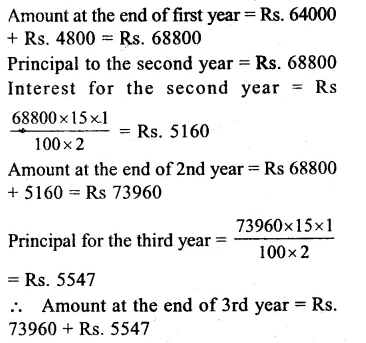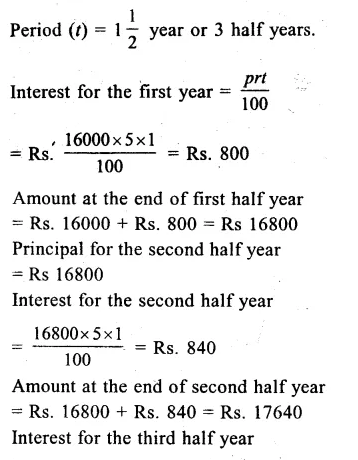## RS Aggarwal Class 8 Solutions Chapter 11 Compound Interest Ex 11A

These Solutions are part of RS Aggarwal Solutions Class 8. Here we have given RS Aggarwal Solutions Class 8 Chapter 11 Compound Interest Ex 11A.

Other Exercises

Question 1.
Solution:
Principal (p) = Rs. 2500
Rate (r) = 10% p.a.
Period (t) = 2 years
Interest for the first year = $$\\ \frac { prt }{ 100 }$$
= $$\\ \frac { 2500X10X1 }{ 100 }$$
= Rs. 250
Amount at the end of first year = Rs. 2500 + Rs. 250
= Rs 2750
Principal for the second year = Rs 2750
Interest for the second year = Rs $$\\ \frac { 2750X10X1 }{ 100 }$$
= Rs. 275
Amount at the end of second year = Rs 2750 + Rs. 275
= Rs. 305
and compound interest for the 2 years = Rs. 3025 – Rs. 2500
= Rs 525 Ans.

Question 2.
Solution:
Principal (P) = Rs. 15625 Rate
(R) = 12% p.a.
Period (n) = 3 yearsQuestion 3.
Solution:
Principal (P) = Rs. 5000
Rate (R) = 9% p.a.
Time (n) = 2 yearsQuestion 4.
Solution:
Amount of loan (p) = Rs. 25000
Rate of interest (r) = 8% p.a.
Period (t) = 2 yearsQuestion 5.
Solution:
In case of Harpreet :
Amount borrowed by Harpreet (P) = Rs. 20000
Rate (r) = 12%
Period (t) = 2 YearsQuestion 6.
Solution:
Principal (p) = Rs. 64000
Rate (r) = $$7\frac { 1 }{ 2 }$$ = $$\\ \frac { 15 }{ 2 }$$%
Period (t) = 3 years
Interest for the first year = $$\\ \frac { prt }{ 100 }$$
= Rs $$\\ \frac { 64000X15X1 }{ 100X2 }$$
= Rs 4800= Rs 79507

Question 7.
Solution:
Principal (p) = Rs 6250
Rate (r) 8% p.a. or 4% half yearly
Period (t) = 1 year = 2 half yearsQuestion 8.
Solution:
Principal (p) = Rs. = 16000
Rate (r) = 10% p.a. or 5% half yearlyHope given RS Aggarwal Solutions Class 8 Chapter 11 Compound Interest Ex 11A are helpful to complete your math homework.

If you have any doubts, please comment below. Learn Insta try to provide online math tutoring for you.